Physics
Easy

Question

# An object is moving at a uniform speed in a counterclockwise, horizontal circle. Which of the following images correctly depicts the force and velocity at a point in time?

##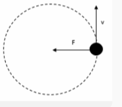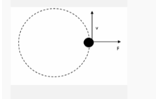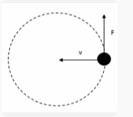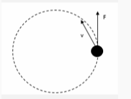Hint:

## The correct answer is:Physics

Physics

Physics

### A boy moves a tied stone above his head in circles, time period of circular motion is 2 sec and it forms a circle of 20 cm radius. What is the speed of rotation?Physics

Physics

Physics

### What is the direction of the velocity at point mentioned if the ball is revolving clockwise.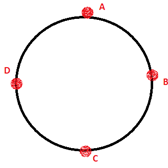At point BPhysics

Physics

Physics

### Which of the following depicts the direction of velocity of the ball circulated around a point?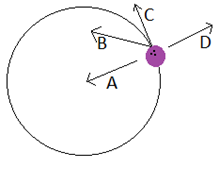Physics

Physics

Physics

### What is the relation between radius, velocity, and time period?Physics

Physics

Physics

### If there is a change in the rate of rotation, then the circular motion will be _______.#### With Turito Foundation.#### Get an Expert Advice From Turito.